Next: Anomalous Dispersion and Resonant Up: Wave Propagation in Uniform Previous: Introduction

# Form of Dielectric Constant

Consider an electromagnetic wave propagating through a transparent, isotropic, dielectric medium. The electric displacement inside the medium is given by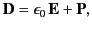(776)

where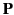is the electric polarization. Because electrons are much lighter than ions (or atomic nuclei), we would expect the former to displace further than the latter under the influence of an electric field. Thus, to a first approximation, the polarization,, is determined by the electron response to the wave. Suppose that the electrons displace an average distance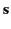from their rest positions in the presence of the wave. It follows that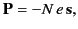(777)

where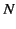is the number density of electrons, and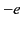the electron charge.

Let us assume that the electrons are bound quasi-elastically'' to their rest positions, so that they seek to return to these positions when displaced from them by an electric field. It follows thatsatisfies a differential equation of the form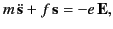(778)

where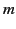is the electron mass,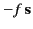is the restoring force, and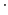denotes a partial derivative with respect to time. The previous equation can also be written(779)

where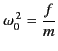(780)

is the characteristic oscillation frequency of the electrons. In almost all dielectric media, this frequency lies in the far ultraviolet region of the electromagnetic spectrum. Note that we have added a phenomenological damping term,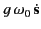, to Equation (780), in order to take into account the fact that an electron excited by an impulsive electric field does not oscillate for ever. In fact, electrons in dielectric media act like high-Q oscillators, which is another way of saying that the dimensionless damping constant,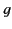, is typically much less than unity. Thus, an electron in a dielectric medium rings'' for a long time after being excited by an electromagnetic impulse.

Let us assume that the electrons oscillate in sympathy with the wave at the wave frequency,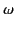. It follows from Equation (780) that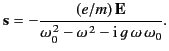(781)

Here, we have neglected the response of the electrons to the magnetic component of the wave. It is easily demonstrated that this is a good approximation provided the electrons do not oscillate with relativistic velocities (i.e., provided the amplitude of the wave is not too large--see Section 7.7). Thus, Equation (778) yields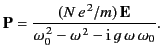(782)

Because, by definition,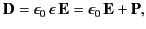(783)

it follows that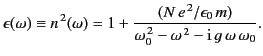(784)

Thus, the index of refraction is indeed frequency dependent. Because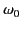typically lies in the ultraviolet region of the spectrum (and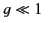), it is clear that the denominator,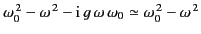, is positive throughout the visible spectrum, and is larger at the red than at the blue end of this spectrum. This implies that blue light is refracted more strongly than red light. This state of affairs, in which higher frequency waves are refracted more strongly than lower frequency waves, is termed normal dispersion. Incidentally, an expression, like the previous one, that (effectively) specifies the phase velocity of waves propagating through a dielectric medium, as a function of their frequency, is usually called a dispersion relation.

Let us now suppose that there aremolecules per unit volume, with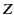electrons per molecule, and that, instead of a single oscillation frequency for all electrons, there are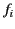electrons per molecule with oscillation frequency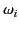and damping constant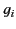. It is easily demonstrated that Equation (785) generalizes to give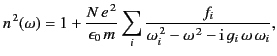(785)

where the oscillator strengths,, satisfy the sum rule,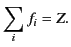(786)

A more exact quantum mechanical treatment of the response of an atom, or molecule, to a low amplitude electromagnetic wave also leads to a dispersion relation of the previous form, except that the quantities,, andcan, in principle, be calculated exactly. In practice, this is too difficult, except in very simple cases.

Because the damping constants,, are generally small compared to unity, it follows from Equation (786) that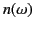is a predominately real quantity at most wave frequencies. The factor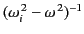is positive for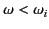, and negative for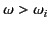. Thus, at low frequencies (i.e., below the smallest) all of the terms appearing in the sum on the right-hand side of (786) are positive, andis consequently greater than unity. Asis raised, such that it exceeds successivevalues, more and more negative terms occur in the sum, until eventually the whole sum is negative, andis less than unity. Hence, at very high frequencies, electromagnetic waves propagate through dielectric media with phase velocities that exceed the velocity of light in a vacuum. For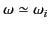, Equation (786) predicts strong variation of the refractive index with frequency. Let us examine this phenomenon more closely.Next: Anomalous Dispersion and Resonant Up: Wave Propagation in Uniform Previous: Introduction
Richard Fitzpatrick 2014-06-27